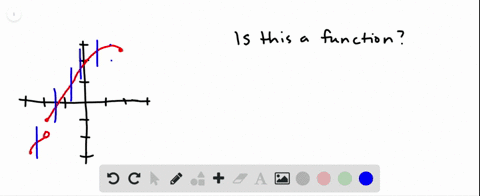Enroll in one of our FREE online STEM summer camps. Space is limited so join now!View Summer Courses### Determine whether the curve is the graph of a fun…

01:38Oregon State University

Need more help? Fill out this quick form to get professional live tutoring.

Get live tutoring
Problem 8

Determine whether the curve is the graph of a function of x. If it is, state the domain and range of the function.

Domain: $x \in[-2,2] ;$ Range: $y \in[-1,2]$

## Discussion

You must be signed in to discuss.

## Video Transcript

when you're looking at a graph. If you want to determine if it's a function, you want to check to see if it passes the vertical line test. Remember, for a function. Every X value must have no more than one y value. So if you pass a vertical line through, you could only go through one point. So if I pass vertical line through lines through at various places here, notice I only go through one point. So yes, this is a function now. What's its domain and what's its range? So for the domain, we want to know what X values it goes through notice. It starts at an X value of negative two and goes over to an X value of two. So the domain in interval notation is negative. 222 That's equivalent to saying negative two is less than or equal to X is less than or equal to two. How about the range? So the range would be the Y values that it's going through. Notice that it's going through a Y value as low as negative one and as high as two and everything in between, so the range would be the interval from negative 1 to 2, and if you writing that as an inequality, it would be negative. One is less than or equal to why is less than or equal to two.